# Case Study Class 10 Maths Questions

Now, CBSE will ask only subjective questions in class 10 Maths case studies. But if you search over the internet or even check many books, you will get only MCQs in the class 10 Maths case study in the session 2022-23. It is not the correct pattern. Just beware of such misleading websites and books.

We advise you to visit CBSE official website (cbseacademic.nic.in) and go through class 10 model question papers. You will find that CBSE is asking only subjective questions under case study in class 10 Maths. We at myCBSEguide helping CBSE students for the past 15 years and are committed to providing the most authentic study material to our students.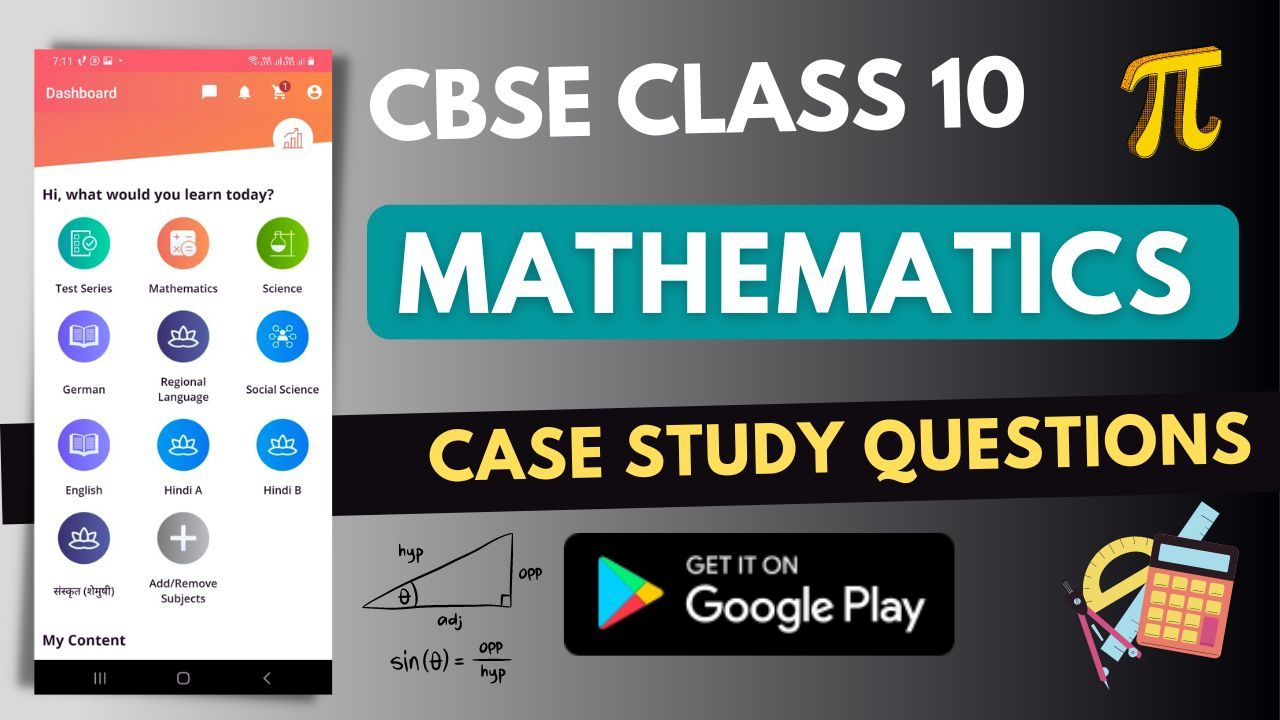Here, myCBSEguide is the only application that has the most relevant and updated study material for CBSE students as per the official curriculum document 2022 – 2023. You can download updated sample papers for class 10 maths.

## Case Study Class 10 Maths Questions

First of all, we would like to clarify that class 10 maths case study questions are subjective and CBSE will not ask multiple-choice questions in case studies. So, you must download the myCBSEguide app to get updated model question papers having new pattern subjective case study questions for class 10 the mathematics year 2022-23.

Class 10 Maths has the following chapters.

• Real Numbers Case Study Question
• Polynomials Case Study Question
• Pair of Linear Equations in Two Variables Case Study Question
• Quadratic Equations Case Study Question
• Arithmetic Progressions Case Study Question
• Triangles Case Study Question
• Coordinate Geometry Case Study Question
• Introduction to Trigonometry Case Study Question
• Some Applications of Trigonometry Case Study Question
• Circles Case Study Question
• Area Related to Circles Case Study Question
• Surface Areas and Volumes Case Study Question
• Statistics Case Study Question
• Probability Case Study Question

## Format of Maths Case-Based Questions

CBSE Class 10 Maths Case Study Questions will have one passage and four questions. As you know, CBSE has introduced Case Study Questions in class 10 and class 12 this year, the annual examination will have case-based questions in almost all major subjects. This article will help you to find sample questions based on case studies and model question papers for CBSE class 10 Board Exams.

## Maths Case Study Question Paper 2023

Here is the marks distribution of the CBSE class 10 maths board exam question paper. CBSE may ask case study questions from any of the following chapters. However, Mensuration, statistics, probability and Algebra are some important chapters in this regard.

 Units Unit Name Marks I NUMBER SYSTEMS 06 II ALGEBRA 20 III COORDINATE GEOMETRY 06 IV GEOMETRY 15 V TRIGONOMETRY 12 V MENSURATION 10 VI STATISTICS & PROBABILITY 11 Total 80

## Case Study Question in Mathematics

Here are some examples of case study-based questions for class 10 Mathematics. To get more questions and model question papers for the 2021 examination, download myCBSEguide Mobile App.

### Case Study Question – 1

In the month of April to June 2022, the exports of passenger cars from India increased by 26% in the corresponding quarter of 2021–22, as per a report. A car manufacturing company planned to produce 1800 cars in 4th year and 2600 cars in 8th year. Assuming that the production increases uniformly by a fixed number every year.Based on the above information answer the following questions.

1. Find the production in the 1st year.
2. Find the production in the 12th year.
3. Find the total production in first 10 years.ORIn which year the total production will reach to 15000 cars?

### Case Study Question – 2

In a GPS, The lines that run east-west are known as lines of latitude, and the lines running north-south are known as lines of longitude. The latitude and the longitude of a place are its coordinates and the distance formula is used to find the distance between two places. The distance between two parallel lines is approximately 150 km. A family from Uttar Pradesh planned a round trip from Lucknow (L) to Puri (P) via Bhuj (B) and Nashik (N) as shown in the given figure below.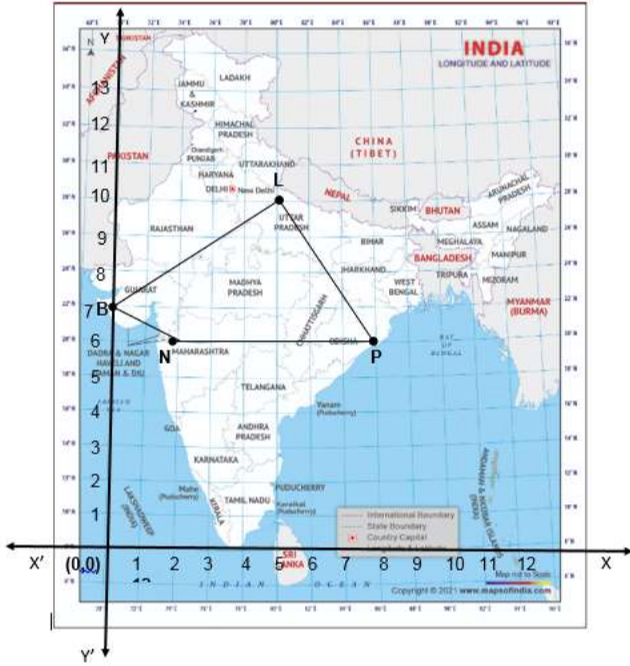Based on the above information answer the following questions using the coordinate geometry.

1. Find the distance between Lucknow (L) to Bhuj(B).
2. If Kota (K), internally divide the line segment joining Lucknow (L) to Bhuj (B) into 3 : 2 then find the coordinate of Kota (K).
3. Name the type of triangle formed by the places Lucknow (L), Nashik (N) and Puri (P)ORFind a place (point) on the longitude (y-axis) which is equidistant from the points Lucknow (L) and Puri (P).

### Case Study Question – 3

Lakshaman Jhula is located 5 kilometers north-east of the city of Rishikesh in the Indian state of Uttarakhand. The bridge connects the villages of Tapovan to Jonk. Tapovan is in Tehri Garhwal district, on the west bank of the river, while Jonk is in Pauri Garhwal district, on the east bank. Lakshman Jhula is a pedestrian bridge also used by motorbikes. It is a landmark of Rishikesh.
A group of Class X students visited Rishikesh in Uttarakhand on a trip. They observed from a point (P) on a river bridge that the angles of depression of opposite banks of the river are 60o and 30o respectively. The height of the bridge is about 18 meters from the river.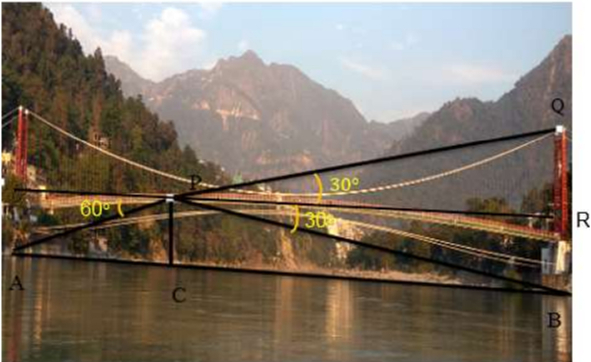Based on the above information answer the following questions.

1. Find the distance PA.
2. Find the distance PB
3. Find the width AB of the river.ORFind the height BQ if the angle of the elevation from P to Q be 30o.

### Case Study Question – 4

A tiling or tessellation of a flat surface is the covering of a plane using one or more geometric shapes, called tiles, with no overlaps and no gaps. Historically, tessellations were used in ancient Rome and in Islamic art. You may find tessellation patterns on floors, walls, paintings etc. Shown below is a tiled floor in the archaeological Museum of Seville, made using squares, triangles and hexagons.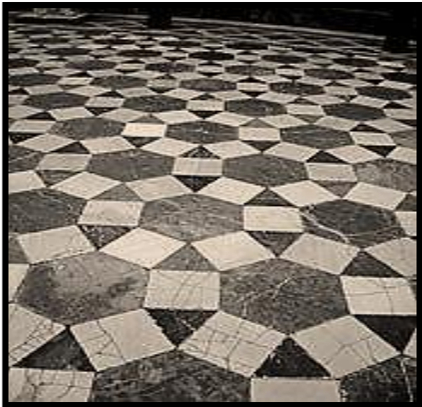A craftsman thought of making a floor pattern after being inspired by the above design. To ensure accuracy in his work, he made the pattern on the Cartesian plane. He used regular octagons, squares and triangles for his floor tessellation pattern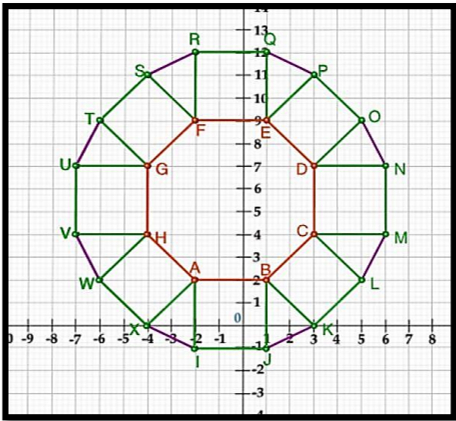Use the above figure to answer the questions that follow:

1. What is the length of the line segment joining points B and F?
2. The centre ‘Z’ of the figure will be the point of intersection of the diagonals of quadrilateral WXOP. Then what are the coordinates of Z?
3. What are the coordinates of the point on y axis equidistant from A and G?ORWhat is the area of area of Trapezium AFGH?

### Case Study Question – 5

The school auditorium was to be constructed to accommodate at least 1500 people. The chairs are to be placed in concentric circular arrangement in such a way that each succeeding circular row has 10 seats more than the previous one.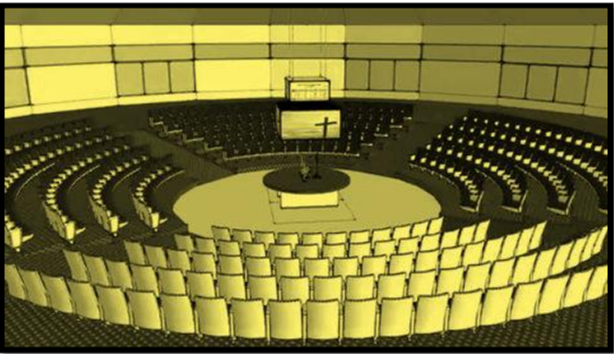1. If the first circular row has 30 seats, how many seats will be there in the 10th row?
2. For 1500 seats in the auditorium, how many rows need to be there?ORIf 1500 seats are to be arranged in the auditorium, how many seats are still left to be put after 10th row?
3. If there were 17 rows in the auditorium, how many seats will be there in the middle row?

### Case Study Question – 6

We all have seen the airplanes flying in the sky but might have not thought of how they actually reach the correct destination. Air Traffic Control (ATC) is a service provided by ground-based air traffic controllers who direct aircraft on the ground and through a given section of controlled airspace, and can provide advisory services to aircraft in non-controlled airspace. Actually, all this air traffic is managed and regulated by using various concepts based on coordinate geometry and trigonometry.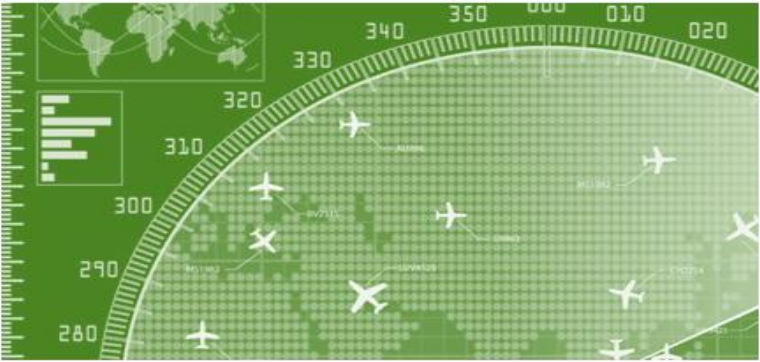At a given instance, ATC finds that the angle of elevation of an airplane from a point on the ground is 60o. After a flight of 30 seconds, it is observed that the angle of elevation changes to 30o. The height of the plane remains constantly asm. Use the above information to answer the questions that follow-

1. Draw a neat labelled figure to show the above situation diagrammatically.
2. What is the distance travelled by the plane in 30 seconds?ORKeeping the height constant, during the above flight, it was observed that after 15(-1) seconds, the angle of elevation changed to 45o. How much is the distance travelled in that duration.
3. What is the speed of the plane in km/hr.

### More Case Study Questions

We have class 10 maths case study questions in every chapter. You can download them as PDFs from the myCBSEguide App or from our free student dashboard.

As you know CBSE has reduced the syllabus this year, you should be careful while downloading these case study questions from the internet. You may get outdated or irrelevant questions there. It will not only be a waste of time but also lead to confusion.

Here, myCBSEguide is the most authentic learning app for CBSE students that is providing you up to date study material. You can download the myCBSEguide app and get access to 100+ case study questions for class 10 Maths.

## How to Solve Case-Based Questions?

Questions based on a given case study are normally taken from real-life situations. These are certainly related to the concepts provided in the textbook but the plot of the question is always based on a day-to-day life problem. There will be all subjective-type questions in the case study. You should answer the case-based questions to the point.

### What are Class 10 competency-based questions?

Competency-based questions are questions that are based on real-life situations. Case study questions are a type of competency-based questions. There may be multiple ways to assess the competencies. The case study is assumed to be one of the best methods to evaluate competencies. In class 10 maths, you will find 1-2 case study questions. We advise you to read the passage carefully before answering the questions.

## Case Study Questions in Maths Question Paper

CBSE has released new model question papers for annual examinations. myCBSEguide App has also created many model papers based on the new format (reduced syllabus) for the current session and uploaded them to myCBSEguide App. We advise all the students to download the myCBSEguide app and practice case study questions for class 10 maths as much as possible.

### Case Studies on CBSE’s Official Website

CBSE has uploaded many case study questions on class 10 maths. You can download them from CBSE Official Website for free. Here you will find around 40-50 case study questions in PDF format for CBSE 10th class.

### 10 Maths Case Studies in myCBSEguide App

You can also download chapter-wise case study questions for class 10 maths from the myCBSEguide app. These class 10 case-based questions are prepared by our team of expert teachers. We have kept the new reduced syllabus in mind while creating these case-based questions. So, you will get the updated questions only.Test Generator

Create question paper PDF and online tests with your own name & logo in minutes.myCBSEguide

Question Bank, Mock Tests, Exam Papers, NCERT Solutions, Sample Papers, Notes